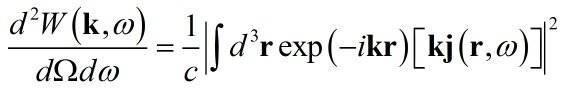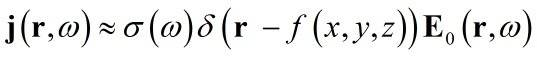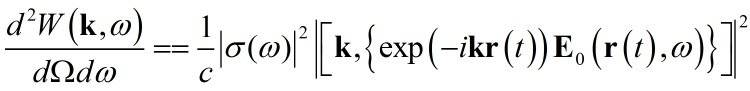# Parametric + analytic function integration

• sukharef

#### sukharef

Hello.
Let's imagine that we have a parametric function f1(x(t),y(t),z(t)) and an analytic one f2(x,y,z) and we have to integrate their product over some volume dx dy dz.
So what are analytical tools for it?
Thanks!

Do you mean that the function f1(x(t),y(t),z(t)) is defined on a curve (x(t),y(t),z(t)) ? The product of f1 and f2 woud only be defined on that curve. So what is your definition of integrating a curve over a volume ?

I think you should give a specific example.

Do you mean that the function f1(x(t),y(t),z(t)) is defined on a curve (x(t),y(t),z(t)) ? The product of f1 and f2 woud only be defined on that curve. So what is your definition of integrating a curve over a volume ?
I think you should give a specific example.
I mean that function f1 represents a curve that can be defined only as parametric. And when i want to calculate an integral f1*f2 over dxdydz i need to turn all my functions into either parametric or into ordinary view as far as i understand. So the question is how can i do it ?
An example can be given as follows:
2d:
f1 = {
x = r1*cos(t)
y = r2*sin(t).
}
f2 = x^2+y^2;
Integral[f1*f2,{x,-1,1}{y,-1,1}];

The integral of the usual sort of curve over an area or volume will be zero because the usual sort of curve has zero area and zero volume.

It would make sense to ask about the integral of the product of two parameterized curves: $\int_{t_0}^{t_1} { f_1(x(t),y(t)) f2(x(t),y(t))} dt.$.

Last edited:
The integral of the usual sort of curve over an area or volume will be zero because the usual sort of curve has zero area and zero volume.

It would make sense to ask about the integral of the product of two parameterized curves: $\int_{t_0}^{t_1} { f_1(x(t),y(t)) f2(x(t),y(t))} dt.$.

To avoid "zero volume" problem let's put f1 into delta-function.
Something like this : DiracDelta[x-x(t)] DiracDelta[y-y(t)] f2 . So after using properties of DiracDelta, integration will be done easily. That's right?

To avoid "zero volume" problem let's put f1 into delta-function.
Something like this : DiracDelta[x-x(t)] DiracDelta[y-y(t)] f2 . So after using properties of DiracDelta, integration will be done easily. That's right?

Using Dirac Delta functions doesn't define a mathematical problem.

For example let $f_2(x,yz)$ to be the constant function $f_2(x,y) = 1$. Let $f_1(x(t),y(t))$ be the line $f_1(x(t),y(t)) = (t,0)$.

Are you asking about whether the notation $\int_0^1 \int_0^1 \delta(x-x(t)) \delta(y-y(t)) (1)\ dy\ dx$ has a mathematical definition? It doesn't. The role of $t$ is unclear.

Using Dirac Delta functions doesn't define a mathematical problem.

For example let $f_2(x,yz)$ to be the constant function $f_2(x,y) = 1$. Let $f_1(x(t),y(t))$ be the line $f_1(x(t),y(t)) = (t,0)$.

Are you asking about whether the notation $\int_0^1 \int_0^1 \delta(x-x(t)) \delta(y-y(t)) (1)\ dy\ dx$ has a mathematical definition? It doesn't. The role of $t$ is unclear.
that's the question, then. parameter t is the only way to define the curve. so what can we do here? we either have to find an analytical way to define the curve without "t" or to find some math tool that modifies the expression that takes into account "t" somehow , i don't know. that's what I'm looking for.

that's the question, then. parameter t is the only way to define the curve. so what can we do here? we either have to find an analytical way to define the curve without "t" or to find some math tool that modifies the expression that takes into account "t" somehow , i don't know. that's what I'm looking for.

The most likely scenario is that you have incorrectly formulated a situation as a mathematical problem.. What situation do you think you are solving by writing down an expression the requires integrating a curve over a volume? For example, if this is supposed to solve a problem in physics, what is the statement of that problem?

The most likely scenario is that you have incorrectly formulated a situation as a mathematical problem.. What situation do you think you are solving by writing down an expression the requires integrating a curve over a volume? For example, if this is supposed to solve a problem in physics, what is the statement of that problem?
the problem is to calculate spectral-angular density ("sad") of radiation produced by electron that moves through spiral curve along it's axis.
so basic expression for "sad" is :, where k - wave vector, j - current density. To make it simple current desity can be represented aswhere G - conductivity, E0 - field of the particle and f(x(t),y(t),z(t)) == r(t) represents the spiral cerve of "zero-volume" (i'm doing it to avoid problem with an object of non-zero volume let's say).
So after this problem of the integration appears:.

As I understand the situation, you have a point charge that moves along a path. It's motion causes an time varying E&M field and you can associate a time varying E&M energy flux with that field. At a given point (x,y,z) in space there is a time varying E&M flux. What is the definition of the radiation density that you want to calculate? Is it an average radiation density - averaged over both space and time ? Or is it a radiation density that is a function of (x,y,z) and time t ?

As I understand the situation, you have a point charge that moves along a path. It's motion causes an time varying E&M field and you can associate a time varying E&M energy flux with that field. At a given point (x,y,z) in space there is a time varying E&M flux. What is the definition of the radiation density that you want to calculate? Is it an average radiation density - averaged over both space and time ? Or is it a radiation density that is a function of (x,y,z) and time t ?
radiation density - at large distances, field energy that contains withing a solid angle - dOmega - , frequency range - domega - , with towards wave vector k. Here "radiation" is diffraction radiation, produced by induced currents that caused by a point charge's field.

The solid angle is referenced to some origin. Is it (0,0,0) ? Because the particle moves, the radiation density within a solid angle referenced to (0,0,0) is not constant in time - is that correct?

The solid angle is referenced to some origin. Is it (0,0,0) ? Because the particle moves, the radiation density within a solid angle referenced to (0,0,0) is not constant in time - is that correct?
Yes. The thing is that we want to see "integrated over time" field energy , so it will be constant in time withing the solid angle.

Then I think the problem has the form:

average radiation density = $\int_{T}\ [\ \int_{(X,Y,Z)} f(x(t),y(t),z(t)) \ dx\ dy\ dz)\ ]\ dt$

The integral $\int_{(X,Y,Z)} f(x(t),y(t),z(t))\ dx\ ,dy\ dz$ is computed regarding $t$ as a constant. This is not the integral of a function along a line. It is the integral of a time varying function defined everywhere within a volume.

Can you compute it as:

average radiation density = $\int_{(X,Y,Z)} \ [ \ \int_{T} f(x(t),y(t),z(t)) dt\ ]\ dx\ dy\ dz$

?

Then I think the problem has the form:

average radiation density = $\int_{T}\ [\ \int_{(X,Y,Z)} f(x(t),y(t),z(t)) \ dx\ dy\ dz)\ ]\ dt$

The integral $\int_{(X,Y,Z)} f(x(t),y(t),z(t))\ dx\ ,dy\ dz$ is computed regarding $t$ as a constant. This is not the integral of a function along a line. It is the integral of a time varying function defined everywhere within a volume.

Can you compute it as:

average radiation density = $\int_{(X,Y,Z)} \ [ \ \int_{T} f(x(t),y(t),z(t)) dt\ ]\ dx\ dy\ dz$

?
i guess it's not an option because $\ \int_{T} f(x(t),y(t),z(t)) dt\$ does not depend on any x,y,z ( x(t) = r1*cos(t), y(t) = ... , z(t) = ... ) so integration over x,y,z in $\int_{(X,Y,Z)} dx\ dy\ dz$ will give us V.

i guess it's not an option because $\ \int_{T} f(x(t),y(t),z(t)) dt\$ does not depend on any x,y,z ( x(t) = r1*cos(t), y(t) = ... , z(t) = ... ) so integration over x,y,z in $\int_{(X,Y,Z)} dx\ dy\ dz$ will give us V.

If we are consider a fixed point in space $(x,y,z)$, the E&M field at that point depends on the distance between the $(x,y,z)$ and the position of the particle $(x_p(t),y_p(t),z_p(t))$ The function we need to integrate can be expressed as $f( x - x_p(t), y - y_p(t), z - z_p(t) )$ so
$\int_T f(x - x_p(t),y - y_p(t), z - z_p(t)) dt = g(x,y,z)$ This is defined for each $(x,y,z)$ in a volume.

If we are consider a fixed point in space $(x,y,z)$, the E&M field at that point depends on the distance between the $(x,y,z)$ and the position of the particle $(x_p(t),y_p(t),z_p(t))$ The function we need to integrate can be expressed as $f( x - x_p(t), y - y_p(t), z - z_p(t) )$ so
$\int_T f(x - x_p(t),y - y_p(t), z - z_p(t)) dt = g(x,y,z)$ This is defined for each $(x,y,z)$ in a volume.
i don'e get it. Parameter t has only one definition - parametric parameter of the curve, spiral curve. Has it anything to do with the position of the particle $(x_p(t),y_p(t),z_p(t))$ ? we have to take into account parametric parameter in the integration over the volume. or maybe I'm wrong?

i don'e get it. Parameter t has only one definition - parametric parameter of the curve, spiral curve.
That is correct. let $t =$ time.

Has it anything to do with the position of the particle $(x_p(t),y_p(t),z_p(t))$ ? we have to take into account parametric parameter in the integration over the volume. or maybe I'm wrong?

Let (x,y,z) be a point in the volume. A point on the path of the electron is $(x_p(t),y_p(t),z_p(t))$.

At each point $(x,y,z)$ the the volume, the radiation field depends on the distance between $(x,y,z)$ and the electron. The radiation field is a function of
$\frac{1}{r^2} = \frac{1}{(x - x_p(t))^2 + (y - y_p(t))^2 + (z - z_p(t))^2}$. So the field and field density is a function F(x,y,z,t).

$\int_T F(x,y,z,t) dt = G(x,y,z)$ There is no variable $t$ in G(x,y,z). We can integrate G(x,y,z) over the volume.

That is correct. let $t =$ time.

Let (x,y,z) be a point in the volume. A point on the path of the electron is $(x_p(t),y_p(t),z_p(t))$.

At each point $(x,y,z)$ the the volume, the radiation field depends on the distance between $(x,y,z)$ and the electron. The radiation field is a function of
$\frac{1}{r^2} = \frac{1}{(x - x_p(t))^2 + (y - y_p(t))^2 + (z - z_p(t))^2}$. So the field and field density is a function F(x,y,z,t).

$\int_T F(x,y,z,t) dt = G(x,y,z)$ There is no variable $t$ in G(x,y,z). We can integrate G(x,y,z) over the volume.
i believe that is not exactly right, because there is also dependence on the path that the electron has covered and this dependence takes into account the spiral curve and it's shape, because it is the source of the radiation we consider.

i believe that is not exactly right, because there is also dependence on the path that the electron has covered.

The dependence on the path the electron has covered is implemented when we do the integration with respect to $t$. Of course you have to know the correct function to integrate with respect to t.

yes, but what about the spiral curve and it's shape?
The dependence on the path the electron has covered is implemented when we do the integration with respect to $t$. Of course you have to know the correct function to integrate with respect to t.
that's right, but i don't have it because field itself is the electron field and radiation field. And to find the last one i have to solve Maxwell's equation first and then do the next step whatever it is. The thing is that to find spectral-angular density i don't have to know radiation field itself, only coordinate dependence.